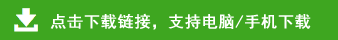# 2020-2021学年上学期二年级数学期中检测卷及参考答案

一、算一算。(12分)

16÷4=  16÷2=　12÷4=　15÷3=
9÷3=    8÷4=     7÷1=    18÷6=
3×5=    4×6=    24+60-7=    2×6-6=
二、填一填。(9分)
1.3+3+3+3+3+3=3×(    )　5+5=(     )×2
2.被除数是4,除数是2,这个算式是(     )。
3.把16个苹果平均分给4个小朋友,每人分得(     )个,如果每人分2个,可以分给(     )人。
4.平行四边形的两组对边长度分别(     )。
5.两个乘数都是5,积是(     );两个加数都是8,和是(     )。
6.把6+6+6+6+6-10改写成乘减算式是(     )。
三、把口诀补充完整。(8分)
二三(      )   四(     )二十四
三(     )十八　  三五(      )
(      )二得四    五五(      )
(      )四得八   四(      )二十
四、选一选。(6分)
四边形(     )　五边形(   　)　六边形(　  )
五、列竖式计算。(16分)
39+28=      83-56=
90-42=　    74-18=
70-24-16=      28+36+19=
71-34+26=     64+17-25=
六、分一分。(6分)
(1)14支铅笔,平均摆成(       )行,每行(       )支。
(2)14支铅笔,每(       )支一行,能摆(        )行。